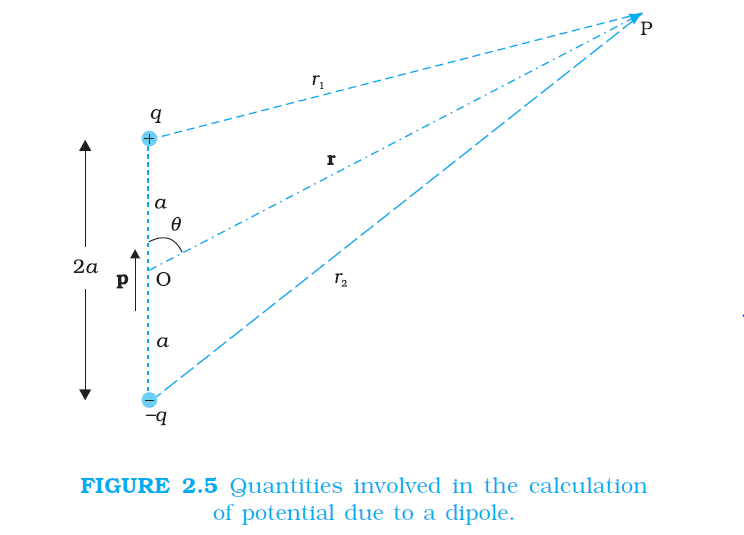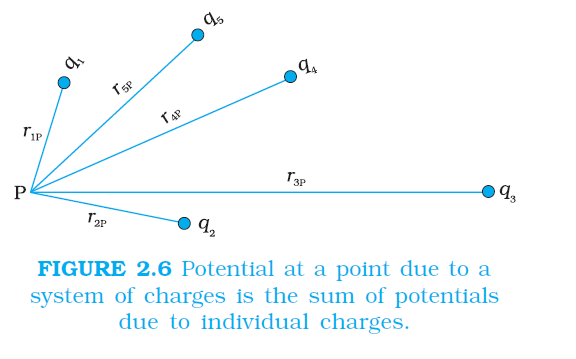Physics POTENTIAL DUE TO AN ELECTRIC DIPOLE , POTENTIAL DUE TO A SYSTEM OF CHARGES FOR CBSE-NCERT 2

### Topic covered

color{blue}{star} POTENTIAL DUE TO AN ELECTRIC DIPOLE
color{blue}{star} POTENTIAL DUE TO A SYSTEM OF CHARGES

### POTENTIAL DUE TO AN ELECTRIC DIPOLEcolor{blue} ✍️ As we learnt in the last chapter, an electric dipole consists of two charges q and –q separated by a (small) distance 2a.

color{blue} ✍️ Its total charge is zero. It is characterised by a dipole moment vector p whose magnitude is q × 2a and which points in the direction from –q to q (Fig. 2.5).

color{blue} ✍️ We also saw that the electric field of a dipole at a point with position vector r depends not just on the magnitude r, but also on the angle between r and p.

color{blue} ✍️ The field falls off, at large distance, not as 1/r^2 (typical of field due to a single charge) but as 1//r^3.

color{blue} ✍️ We, now, determine the electric potential due to a dipole and contrast it with the potential due to a single charge. As before, we take the origin at the centre of the dipole.

color{blue} ✍️ Now we know that the electric field obeys the superposition principle. Since potential is related to the work done by the field, electrostatic potential also follows the superposition principle. Thus, the potential due to the dipole is the sum of potentials due to the charges q and –q.

color{blue}(V = 1/(4piepsilon_0) (q/r_1-q/r_2)) .................2.9

color{blue} ✍️ where r_1 and r_2 are the distances of the point P from q and –q, respectively.

Now, by geometry,

color{purple}(r_(1)^(2) = r^2 + a^2 − 2ar cosθ)

color{purple}(r_(2)^(2) = r^2 + a^2 + 2ar cosθ) ................2.10

color{blue} ✍️ We take r much greater than a (r >> a ) and retain terms only upto the first order in a/r

color{blue} ✍️ We take r much greater than a ( r >> a ) and retain terms only upto the first order in a//r

color{purple}(≅ r^2 (1 -(2acos theta) + (a^2/r^2)))

color{purple}(≅ r^2 (1 - (2acos theta)/r)) ....................2.11

Similarly,

color{purple}(r_(2)^(2) = r^2 (1 + (2acos theta)/r)) .........................2.12

color{blue} ✍️ Using the Binomial theorem and retaining terms upto the first order in a//r ; we obtain,

color{purple}(1/(r_1) ≅ 1/r (1 -(2acos theta)/r)^(-1//2) ≅ 1/r (1+a/r cos theta)) ....... 2.13 (a)

color{purple}(1/(r_2) ≅ 1/r (1 + (2acos theta)/r)^(-1//2) ≅ 1/r (1 - a/r cos theta)) ..............2.13(b)

color{blue} ✍️ Using Eqs. (2.9) and (2.13) and p = 2qa, we get

color{purple}(V = q/(4piepsilon_0) (2acostheta)/(r^2) = (pacostheta)/(4pi epsilon_0r^2)) .................... 2.14

Now, color{purple}{p cos (θ = p*hat r)}

where hat r is the unit vector along the position vector OP. The electric potential of a dipole is then given by

color{blue}(V = 1/(4piepsilon_0) (p*hatr)/(r^2) : (r > > a))

.................2.15

color{blue} ✍️ Equation (2.15) is, as indicated, approximately true only for distances large compared to the size of the dipole, so that higher order terms in a/r are negligible. For a point dipole p at the origin, Eq. (2.15) is, however, exact.

color{blue} ✍️ From Eq. (2.15), potential on the dipole axis (θ = 0, π ) is given by

color{blue}(V= ± 1/(4piepsilon_0) p/(r^2))

.....................2.16

color{blue} ✍️ Positive sign for θ = 0, negative sign for θ = π.) The potential in the equatorial plane (θ = π//2) is zero. The important contrasting features of electric potential of a dipole from that due to a single charge are clear from Eqs. (2.8) and (2.15):

color{blue} ✍️ The potential due to a dipole depends not just on r but also on the angle between the position vector r and the dipole moment vector p. (It is, however, axially symmetric about p. That is, if you rotate the position vector r about p, keeping θ fixed, the points corresponding to P on the cone so generated will have the same potential as at P.)

color{blue} ✍️ The electric dipole potential falls off, at large distance, as 1//r^2, not as 1//r, characteristic of the potential due to a single charge. (You can refer to the Fig. 2.5 for graphs of 1//r^2 versus r and 1//r versus r, drawn there in another context.)

### POTENTIAL DUE TO A SYSTEM OF CHARGEScolor{blue} ✍️ Consider a system of charges q_1, q_2,…, qn with position vectors r_1, r_2,…, r_n relative to some origin (Fig. 2.6). The potential V_1 at P due to the charge q_1 is

color{purple}(V = 1/(4piepsilon_0) (q_1)/(r_(1P)))

color{blue} ✍️ where r_(1P) is the distance between q_1 and P. Similarly, the potential V_2 at P due to q_2 and V_3 due to q_3 are given by

color{purple}(V = 1/(4piepsilon_0) (q_2)/(r_(2P)) ; V = 1/(4piepsilon_0) (q_3)/(r_(3P)))

color{blue} ✍️ where r_(2P) and r_(3P) are the distances of P from charges q_2 and q_3, respectively; and so on for the potential due to other charges. By the superposition principle, the potential V at P due to the total charge configuration is the algebraic sum of the potentials due to the individual charges

color{purple}(V = V_1 + V_2 + .... V_n) ..........2.17

color{red}{V = 1/(4piepsilon_0) [ (q_1)/(r_(1P)) +((q_2))/(r_(2P)) + ....... + (q_n)/(r_(nP))]

.....................2.18

color{blue} ✍️ If we have a continuous charge distribution characterised by a charge density ρ (r), we divide it, as before, into small volume elements each of size Δv and carrying a charge ρΔv.

color{blue} ✍️ We then determine the potential due to each volume element and sum (strictly speaking , integrate) over all such contributions, and thus determine the potential due to the entire distribution.

color{blue} ✍️ We have seen in Chapter 1 that for a uniformly charged spherical shell, the electric field outside the shell is as if the entire charge is concentrated at the centre. Thus, the potential outside the shell is given by

color{red}(V = 1/(4piepsilon_0) p/r \ \ \ \ (r >= R)) ...............................2.19(a)

color{blue} ✍️ where q is the total charge on the shell and R its radius. The electric field inside the shell is zero.

color{blue} ✍️ This implies (Section 2.6) that potential is constant inside the shell (as no work is done in moving a charge inside the shell), and, therefore, equals its value at the surface, which is

color{purple}(V = 1/(4piepsilon_0) p/R) ............................2.19 (b)

Q 3114580459Two charges 3 xx 10^(–8) C and –2 xx 10^(–8) C are located
15 cm apart. At what point on the line joining the two charges is the
electric potential zero? Take the potential at infinity to be zero.
Class 12 Chapter 2 Example 2Solution:

Let us take the origin O at the location of the positive charge. The line joining the two charges is taken to be the x-axis; the negative charge is taken to be on the right side of the origin (Fig. 2.7). Let P be the required point on the x-axis where the potential is zero. If x is the x-coordinate of P, obviously x must be positive. (There is no possibility of potentials due to the two charges adding up to zero for x < 0.) If x lies between O and A, we have

color{blue}{1/(4piepsilon_0) [ (3xx10^(-8))/(x-10^(-2)) - (2xx10^(-8))/((15-x)xx10^(-2)] ]= 0}

where x is in cm. That is,

3/x - 2 /(15-x)=0

which gives x = 9 cm.

If x lies on the extended line OA, the required condition is

3/x - (2)/(x-15) = 0

which gives
x = 45 cm
Thus, electric potential is zero at 9 cm and 45 cm away from the
positive charge on the side of the negative charge. Note that the
formula for potential used in the calculation required choosing
potential to be zero at infinity.
Q 3134680552Figures 2.8 (a) and (b) show the field lines of a positive and negative point charge respectively.

a) Give the signs of the potential difference V_P – V_Q; V_B – V_A.
(b) Give the sign of the potential energy difference of a small negative charge between the points Q and P; A and B.
(c) Give the sign of the work done by the field in moving a small positive charge from Q to P.
(d) Give the sign of the work done by the external agency in moving a small negative charge from B to A.
(e) Does the kinetic energy of a small negative charge increase or decrease in going from B to A?
Class 12 Chapter 2 Example 3Solution:

(a) as V prop 1/r, V_p > V_Q Thus (V_P-V_Q) is positive. Also V_B is less negative than V_A . Thus, V_B > V_A or (V_B – V_A) is positive.

(b) A small negative charge will be attracted towards positive charge.
The negative charge moves from higher potential energy to lower potential energy. Therefore the sign of potential energy difference of a small negative charge between Q and P is positive. Similarly, (P.E.)A > (P.E.)B
and hence sign of potential energy differences is positive.
(c) In moving a small positive charge from Q to P, work has to be done by an external agency against the electric field. Therefore, work done by the field is negative.
(d) In moving a small negative charge from B to A work has to be done by the external agency. It is positive.
(e) Due to force of repulsion on the negative charge, velocity decreases and hence the kinetic energy decreases in going from B to A.